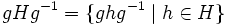# Equivalence of conjugacy and coset definitions of normality

This article gives a proof/explanation of the equivalence of multiple definitions for the term normal subgroup
View a complete list of pages giving proofs of equivalence of definitions
This article gives a proof/explanation of the equivalence of multiple definitions for the term normalizer
View a complete list of pages giving proofs of equivalence of definitions

## Statement

The following are equivalent for a subgroup$H$ of a group$G$ and an element$g \in G$:

1.$gHg^{-1} = H$. Here,$gHg^{-1} = \{ ghg^{-1} \mid h \in H \}$ is the conjugate subgroup of$H$ by$g$.
2.$gH = Hg$. Here,$gH = \{ gh \mid h \in H\}$ is the Left coset (?) of$g$ for the subgroup$H$ and$Hg = \{ hg \mid h \in H \}$ is the right coset of$g$ for the subgroup$H$.

The set of$g \in G$ such that the equivalent conditions (1) and (2) hold is termed the Normalizer (?) of$H$ in$G$.$H$ is a Normal subgroup (?) of$G$ if the above two conditions hold for all$g \in G$.

## The techniques used in this proof

For a survey article describing these techniques in more detail, see manipulating equations in groups.

## Proof

### (1) implies (2)

Given:$H$ a subgroup of$G$,$g \in G$, and$gHg^{-1} = H$.

To prove:$gH = Hg$.$gHg^{-1} = H$

and multiply both on the right by$g$. We get:$gHg^{-1}g = Hg$

which simplifies to:$gH = Hg$.

A clearer justification of the manipulation done on the left side can be obtained by looking at things elementwise. We have$gHg^{-1} = \{ ghg^{-1}\mid h \in H \}$. Thus,$(gHg^{-1})g = \{ (ghg^{-1})g \mid h \in H \} = \{ hg : h \in H\}$.

### (2) implies (1)

Given:$H$ a subgroup of$G$,$g \in G$, and$gH = Hg$.

To prove:$gHg^{-1} = H$.$gH = Hg$
We multiply both sides on the right by$g^{-1}$, and obtain:$gHg^{-1} = Hgg^{-1}$$gHg^{-1} = H$.
A clearer justification of the manipulation done on the right side can be obtained by looking at things elementwise. We have$Hg = \{ hg \mid h \in H \}$ and$(Hg)g^{-1} = \{ (hg)g^{-1} \mid h \in H \} = \{ h \mid h \in H \} = H$.